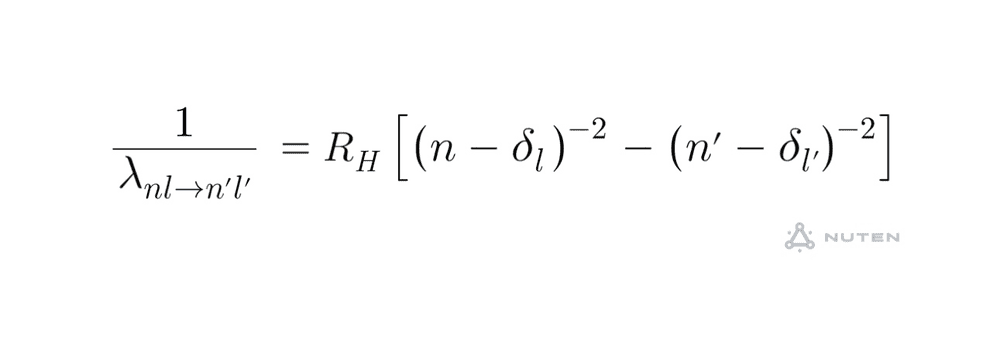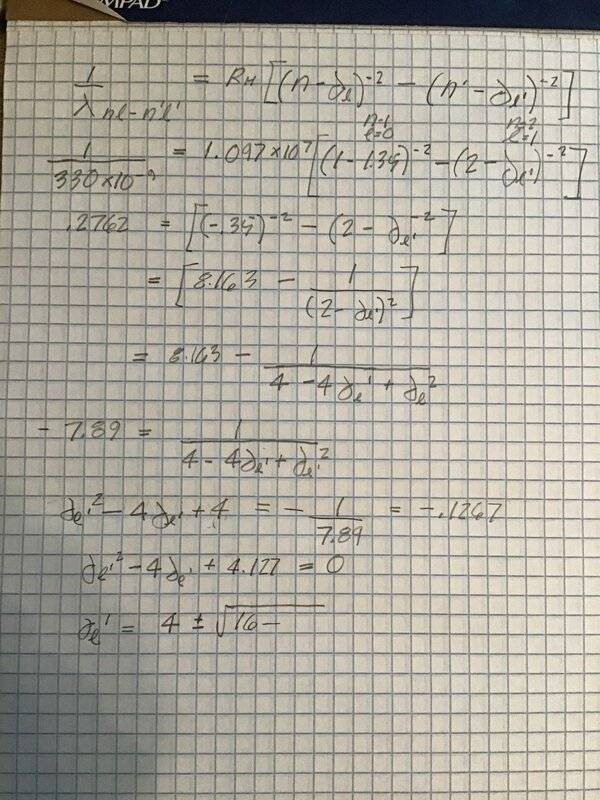# Quantum Defect

jjson775
Homework Statement:
When the outermost electron of an alkali atom is excited, it is found that states with the same n but different l have slightly different energies because they penetrate into the core to different degrees. Low l orbitals penetrate more, while higher l orbitals penetrate less. The wavelengths of absorption lines are given approximately by the equation below.

Observe that it is like the equation that describes hydrogen but with the principal quantum numbers replaced by effective quantum numbers. Here delta l is the “quantum defect” associated with orbital quantum number l. The value of delta l is independent of n. For sodium Na delta 0 = 1.35. The longest wavelength for an absorption transition carrying Na from its ground state to a state with higher principal quantum number is 330 no. A) For what other value of l can you determine the quantum defect and B) what is the value of that defect.
Relevant Equations:
See belowHomework Helper
Gold Member
What is the value of n for the outer electron in the ground state?

Looks like you dropped a negative sign about halfway through your calculation.

You can avoid solving a general quadratic equation by letting ##x = n'-\delta_{l'}## and first solving for ##x##.

Last edited:
jjson775
That’s it, a simple solution. Thanks